×#### Thank you for registering.

One of our academic counsellors will contact you within 1 working day.

Click to Chat

1800-1023-196

+91-120-4616500

CART 0

• 0

MY CART (5)

Use Coupon: CART20 and get 20% off on all online Study Material

ITEM
DETAILS
MRP
DISCOUNT
FINAL PRICE
Total Price: Rs.

There are no items in this cart.
Continue Shopping• Complete JEE Main/Advanced Course and Test Series
• OFFERED PRICE: Rs. 15,900
• View Details

```Revision Notes on Chemical Reactions and Equations

Chemical Reactions and Equations

Any process that involves the rearrangement of structure of the substance or conversion of reactants into products is defined as Chemical Reaction.

For a Chemical Reaction to occur, the change can be observed in the form of -

Change in State: Melting of ice into water.

Change in Colour: Iron rusting which has colour change from silver to reddish brown.Change in Temperature: There are two types of reaction i.e Exothermic and Endothermic Reaction.

Exothermic Reactions: Those reactions in which energy is released in the form of heat are called Exothermic Reactions.

Examples -

(1) All combustion reactions e.g.

CH4+ 2O2 —> CO2 + 2H2O + Heat

(2) Thermite reactions e.g.

2A1 + Fe2O3 —> 2Fe + Al2O3 + Heat

Combinations are generally exothermic in nature. The decomposition of organic matters into compost is an example of exothermic reaction.

Endothermic Reactions: Those reactions in which energy is absorbed are called Endothermic Reactions.

Examples -also, the reaction of photosynthesis -Evolution of any gas: When Zinc reacts with sulphuric acid it gives hydrogen gas.

Zn + H2 SO4­ → ZnSO4 + H2

Formation of Precipitate: When a soluble carbonate reacts with Barium, Barium Carbonate precipitate can be observed.

Change in State

Some chemical reactions are characterized by a change in state.

When wax is burned (in the form of wax candle,) then water and carbon dioxide are formed.

Now, wax is a liquid whereas carbon dioxide is a gas. This means that during the combustion reaction of wax, the physical state changes from solid to liquid and gas.

Physical Change

In this change identity of the substance remains same.

For Example, Melting, Boiling etc.

Chemical Change

The identity of the substances change

Reactants are converted into substance due to formation or broken down of older bonds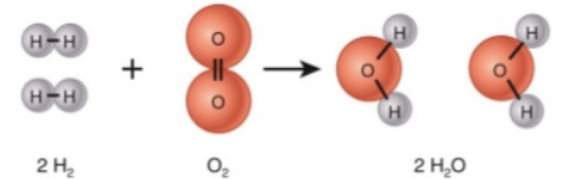Chemical Equation

The symbolic representation of chemical reaction using symbols and formulae is known as Chemical Equation. For this, reactants are written in left hand side whereas products are written on the right.

Balanced Chemical Equation

A balanced chemical equation is the one where the number of atoms involved in reactants side is equal to number of atoms on product side.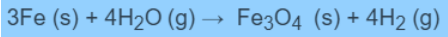Eq.1. Example of Balanced Chemical Equation

Steps to form Balanced Equation

To show how to balance the equation, the following equation is used-

Fe + H2O → Fe3O4 + H2

Step 1: First of all, draw the boxes around each formula as shown below-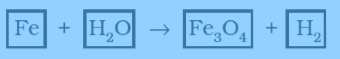Step 2: Find out the number of atoms of each element. For Example, on reactant side, 1 for Fe, 2 H, and 1 O and on product side we have, 3 for Fe, 4 for O and 2 for H.

Step 3: Start to balance the equation with the compound having maximum number of atoms. While balancing does not alter the formula of the compound.

Step 4: One by one balance each element on reactant and product side.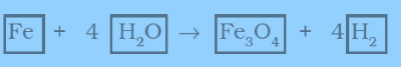Step 5: After balancing number of atoms on both the side of the equation, finally check the correctness of the balanced equation.Step 6: then write the symbols of the physical state of reactants and products as shown below-

3Fe(s) + 4H2O (g) → Fe3O4 (s) + 4H2 (g)

This above equation represents the balanced equation.

Balancing a Redox Reaction

The basic ionic form of the equation is-

Fe2+ + Cr2O72- → Fe3+ + Cr3+

Oxidation half reaction is-Reduction half reaction is-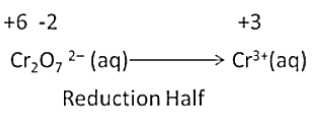Use the reduction half method to balance the equation. Balance the atoms in each half of the reaction except H and O atoms.

Cr2O72- (aq) → 2 Cr3+(aq)

Add water molecules as the reaction is taking place in acidic solution. This is to balance the O atoms and hydrogen ions.

Cr2O72- (aq) + 14 H+(aq) → 2 Cr3+(aq) + 7H2O  (I)

Then balance the charges in both half reactions.

Fe2+(aq) → Fe3+(aq) + e-

Cr2O72- (aq) + 14 H+ + 6e- → 2 Cr3+ + 7H2O

6 Fe2+(aq) → 6 Fe3+(aq) + 6e-

Two half of the equations are added to get the overall reaction

6Fe2+(aq) + Cr2O72-(aq) + 14H+(aq) → 6Fe3+(aq) + 2Cr3+(aq) + 7H2O (I)

Types of Chemical Reaction

Combination Reaction is reaction when single product is formed from the combination of two or more reactants. For Example-

​Eq.2. Example of Combination Reaction

Reactions can be exothermic as well as endothermic. Exothermic reaction release heats and raises the temperature of the surroundings. For Example, Respiration is an example of exothermic reaction.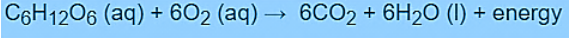Eq.3. Example of Exothermic Reaction

Endothermic reaction involved the absorption of the heat and thus it cools the surrounding. The decomposition of dead organic material is an endothermic reaction.

Decomposition Reaction is type of reaction which involves breakdown of single reactant into simpler products. Decomposition of silver chloride into silver and chlorine in presence of sunlight is an example of decomposition reaction.Eq.4. Example of Decomposition Reaction

Displacement Reaction is a reaction in which more reactive element will displaces the less reactive element.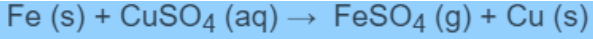Eq. 5. Example of Displacement Reaction

Double Displacement Reaction is a type of reaction in which cations and anions in the reactants switch the places to form new products.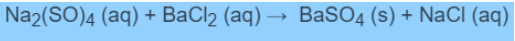Eq. 6. Example of Double Displacement Reaction

Redox Reaction is also known as Oxidation-reduction Reaction. In this type of reaction transfer of electrons occurs between the two species. Oxidation is defined as addition of oxygen or removal of hydrogen. Reduction is defined as removal of oxygen or addition of hydrogen. Oxidizing agent is the one which gains the electrons and is reduced in a chemical reaction. Reducing agent is oxidized in a chemical reaction and it loses the electrons. Fluorine is the strongest oxidizing agent. Formic acid is a reducing agent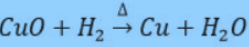Eq.7. Example of Redox Reaction

Corrosion

Metals are prone to corrosion. It is a slow conversion of metals into some undesirable compounds. This occur may be due to reaction with oxygen, gases, acids etc. When irons reacts with atmospheric oxygen and moisture, a red layer is formed on the surface of the iron, this process is known as Rusting.Eq. 8. Equation for Iron Rusting

Rancidity

When food containing fats and oils are exposed to the atmosphere, the oxidation of fat and oil occurs, this is known as Rancidity.

Methods to Prevent Rancidity

Store cooking oils from direct sunlight.

Food should be placed at low temperature.

By adding antioxidants food can be protected from rancidity.

Packing material should replace the air with nitrogen.

Minimize the use of salts in fried foods.

```### Course Features

• 728 Video Lectures
• Revision Notes
• Previous Year Papers
• Mind Map
• Study Planner
• NCERT Solutions
• Discussion Forum
• Test paper with Video Solution